# Secretary Problem (A Optimal Stopping Problem)

The Secretary Problem also known as marriage problem, the sultan’s dowry problem, and the best choice problem is an example of Optimal Stopping Problem. This problem can be stated in the following form: Imagine an administrator who wants to hire the best secretary out of n rankable applicants for a position. The applicants are interviewed one by one in random order. A decision about each particular applicant is to be made immediately after the interview. Once rejected, an applicant cannot be recalled. During the interview, the administrator can rank the applicant among all applicants interviewed so far but is unaware of the quality of yet unseen applicants. The question is about the optimal strategy (stopping rule) to maximize the probability of selecting the best applicant. Optimal Stopping : In mathematics, the theory of optimal stopping or early stopping is concerned with the problem of choosing a time to take a particular action, in order to maximize an expected reward or minimize an expected cost. If the decision to hire an applicant was to be taken in the end of interviewing all the n candidates, a simple solution is to use maximum selection algorithm of tracking the running maximum (and who achieved it) and selecting the overall maximum at the end. The difficult part of this problem is that the decision must be made immediately after interviewing a candidate. 1/e law of Optimal Strategy According to this strategy, the optimal win probability is always at least 1/e. The optimal stopping rule prescribes always rejecting the first n/e applicants that are interviewed (where e is the base of the natural logarithm and has the value 2.71828) and then stopping at the first applicant who is better than every applicant interviewed so far (or continuing to the last applicant if this never occurs). This strategy is called the 1/e stopping rule because the probability to select the best candidate is 1/e, in other words, this strategy selects the best candidate about 37% of time. If you think carefully, it might seem obvious that one cannot select the first candidate because the first candidate has no one to compare with. A better strategy is to choose a few candidates as a sample to set the benchmark for remaining candidates. So the sample will be rejected and will only be used for setting benchmark.

• If a sample is too small, we don’t get information enough for setting the benchmark for remaining candidates.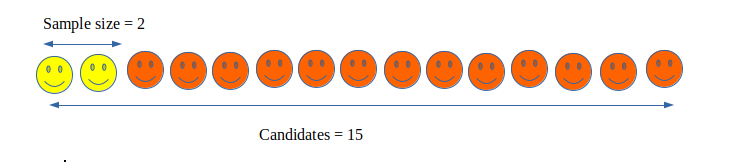• If a sample is too large, though we get plenty of information but we have also burned too many of the potential candidates. This leaves us with very few candidates to choose from, and hence making the strategy a poor one.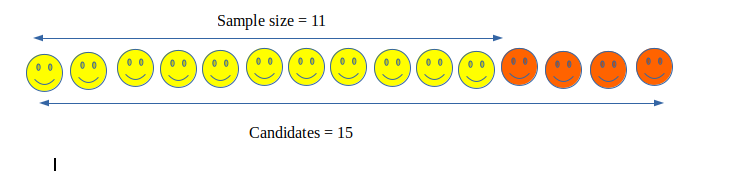• The best strategy is to choose the perfect or optimal sample size (ideal sample size) which can be done using 1/e law that is rejecting n/e candidates (this n/e is the sample size).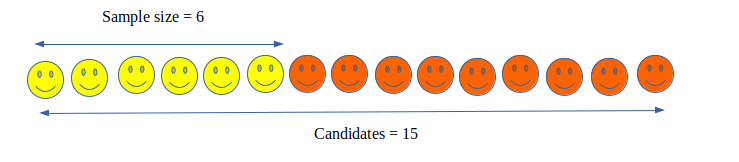The optimal sample size and Probability of success for different values of n are :
Optimal Sample size k = n / e
Probability of success is given by :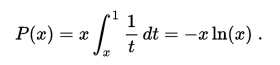where x = k / n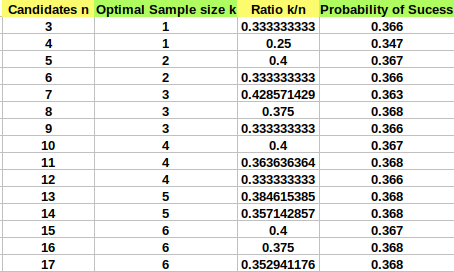The probability of selecting the best applicant in the classical secretary problem converges toward 1/e = 0.368 (approx)

Program to Test Secretary Problem :
Note* : The Optimal Strategy doesn’t always find the best candidate but selects the almost best candidates most of the times.

## C++

 `// C++ Program to test 1/e law for Secretary Problem :` `#include ` `#include ` `#define e 2.71828` `using` `namespace` `std;` `  `  `// To find closest integer of num.` `int` `roundNo(``float` `num)` `{` `    ``return` `num < 0 ? num - 0.5 : num + 0.5;` `}` `  `  `// Finds best candidate using n/e rule. candidate[]` `// represents talents of n candidates.` `void` `printBestCandidate(``int` `candidate[], ``int` `n)` `{` `    ``// Calculating sample size for benchmarking.` `    ``int` `sample_size = roundNo(n/e);` `    ``cout << ``"\n\nSample size is "` `<< sample_size << endl;` `  `  `    ``// Finding best candidate in sample size` `    ``int` `best = 0; ` `    ``for` `(``int` `i = 1; i < sample_size; i++)` `        ``if` `(candidate[i] > candidate[best])` `            ``best = i;` `  `  `    ``// Finding the first best candidate that is  ` `    ``// better than benchmark set.` `    ``for` `(``int` `i = sample_size; i < n; i++)` `        ``if` `(candidate[i] >= candidate[best]) {` `            ``best = i;` `            ``break``;` `        ``}` `  `  `    ``if` `(best >= sample_size)` `        ``cout << endl << ``"Best candidate found is "` `            ``<< best + 1 << ``" with talent "` `            ``<< candidate[best] << endl;` `    ``else` `        ``cout << ``"Couldn't find a best candidate\n"``;` `}` `  `  `int` `main()` `{` `    ``int` `n = 8;` `  `  `    ``// n = 8 candidates and candidate array contains` `    ``// talents of n candidate where the largest ` `    ``// number means highest talented candidate.` `    ``int` `candidate[n];` `  `  `    ``// generating random numbers between 1 to 8 ` `    ``// for talent of candidate` `    ``srand``(``time``(0));    ` `    ``for` `(``int` `i = 0; i < n; i++)` `        ``candidate[i] = 1 + ``rand``() % 8;` `  `  `    ``cout << ``"Candidate : "``;` `    ``for` `(``int` `i = 0; i < n; i++)` `        ``cout << i + 1 << ``" "``;` `    ``cout << endl;` `    ``cout << ``"  Talents : "``;` `    ``for` `(``int` `i = 0; i < n; i++)` `        ``cout << candidate[i] << ``" "``;` `      `  `    ``printBestCandidate(candidate, n);` `  `  `    ``return` `0;` `}`

## Java

 `// Java Program to test 1/e law for Secretary Problem :` `import` `java.util.*;` `class` `GFG` `{` `static` `double` `e = ``2.71828``;` `  `  `// To find closest integer of num.` `static` `int` `roundNo(``float` `num)` `{` `    ``return` `(``int``) (num < ``0` `? ` `                  ``num - ``0.5` `: num + ``0.5``);` `}` `  `  `// Finds best candidate using n/e rule. candidate[]` `// represents talents of n candidates.` `static` `void` `printBestCandidate(``int` `candidate[], ``int` `n)` `{` `    ``// Calculating sample size for benchmarking.` `    ``int` `sample_size = roundNo((``float``) (n / e));` `    ``System.out.println(``"\n\nSample size is "` `+ sample_size);` `  `  `    ``// Finding best candidate in sample size` `    ``int` `best = ``0``; ` `    ``for` `(``int` `i = ``1``; i < sample_size; i++)` `        ``if` `(candidate[i] > candidate[best])` `            ``best = i;` `  `  `    ``// Finding the first best candidate that is ` `    ``// better than benchmark set.` `    ``for` `(``int` `i = sample_size; i < n; i++)` `        ``if` `(candidate[i] >= candidate[best])` `        ``{` `            ``best = i;` `            ``break``;` `        ``}` `  `  `    ``if` `(best >= sample_size)` `        ``System.out.println(``"\nBest candidate found is "` `+ ` `                           ``(best + ``1``) + ``" with talent "` `+ ` `                            ``candidate[best]);` `    ``else` `        ``System.out.print(``"Couldn't find a best candidate\n"``);` `}` `  `  `// Driver Code` `public` `static` `void` `main(String[] args)` `{` `    ``int` `n = ``8``;` `  `  `    ``// n = 8 candidates and candidate array contains` `    ``// talents of n candidate where the largest ` `    ``// number means highest talented candidate.` `    ``int` `[]candidate = ``new` `int``[n];` `  `  `    ``// generating random numbers between 1 to 8 ` `    ``// for talent of candidate` `    ``Random rand = ``new` `Random();` `    ``for` `(``int` `i = ``0``; i < n; i++)` `        ``candidate[i] = ``1` `+ rand.nextInt((``8` `- ``1``) + ``1``);` `  `  `    ``System.out.print(``"Candidate : "``);` `    ``for` `(``int` `i = ``0``; i < n; i++)` `        ``System.out.print(i + ``1` `+ ``" "``);` `    ``System.out.println();` `    ``System.out.print(``"Talents : "``);` `    ``for` `(``int` `i = ``0``; i < n; i++)` `        ``System.out.print(candidate[i] + ``" "``);` `      `  `    ``printBestCandidate(candidate, n);` `}` `}` `  `  `// This code is contributed by 29AjayKumar`

## Python3

 `# Python3 Program to test 1/e law for` `# Secretary Problem` `import` `random` `import` `math` `  `  `e ``=` `2.71828``;` `  `  `# To find closest integer of num.` `def` `roundNo(num):` `    ``if``(num < ``0``): ` `        ``return` `(num ``-` `0.5``)` `    ``else``: ` `        ``return` `(num ``+` `0.5``);` `  `  `# Finds best candidate using n/e rule. ` `# candidate[] represents talents of n candidates.` `def` `printBestCandidate(candidate, n):` `      `  `    ``# Calculating sample size for benchmarking.` `    ``sample_size ``=` `roundNo(n ``/` `e);` `    ``print``(``"\n\nSample size is"``, ` `           ``math.floor(sample_size));` `  `  `    ``# Finding best candidate in sample size` `    ``best ``=` `0``; ` `    ``for` `i ``in` `range``(``1``, ``int``(sample_size)):` `        ``if` `(candidate[i] > candidate[best]):` `            ``best ``=` `i;` `  `  `    ``# Finding the first best candidate that ` `    ``# is better than benchmark set.` `    ``for` `i ``in` `range``(``int``(sample_size), n):` `        ``if` `(candidate[i] >``=` `candidate[best]):` `            ``best ``=` `i;` `            ``break``;` `  `  `    ``if` `(best >``=` `int``(sample_size)):` `        ``print``(``"\nBest candidate found is"``, ` `                     ``math.floor(best ``+` `1``), ` `              ``"with talent"``, math.floor(candidate[best]));` `    ``else``:` `        ``print``(``"Couldn't find a best candidate"``);` `  `  `# Driver code` `n ``=` `8``;` `  `  `# n = 8 candidates and candidate ` `# array contains talents of n ` `# candidate where the largest ` `# number means highest talented ` `# candidate.` `candidate ``=` `[``0``] ``*` `(n);` `  `  `# generating random numbers between 1 to 8 ` `# for talent of candidate` `for` `i ``in` `range``(n):` `    ``candidate[i] ``=` `1` `+` `random.randint(``1``, ``8``);` `print``(``"Candidate : "``, end ``=` `"");` `  `  `for` `i ``in` `range``(n):` `    ``print``((i ``+` `1``), end ``=` `" "``);` `print``(``"\nTalents : "``, end ``=` `"");` `  `  `for` `i ``in` `range``(n):` `    ``print``(candidate[i], end ``=` `" "``);` `   `  `printBestCandidate(candidate, n);` `  `  `# This code is contributed by mits`

## C#

 `// C# Program to test 1/e law for Secretary Problem` `using` `System;` `      `  `class` `GFG` `{` `static` `double` `e = 2.71828;` `  `  `// To find closest integer of num.` `static` `int` `roundNo(``float` `num)` `{` `    ``return` `(``int``) (num < 0 ? ` `                  ``num - 0.5 : num + 0.5);` `}` `  `  `// Finds best candidate using n/e rule. candidate[]` `// represents talents of n candidates.` `static` `void` `printBestCandidate(``int` `[]candidate, ``int` `n)` `{` `    ``// Calculating sample size for benchmarking.` `    ``int` `sample_size = roundNo((``float``) (n / e));` `    ``Console.WriteLine(``"\n\nSample size is "` `+ ` `                                ``sample_size);` `  `  `    ``// Finding best candidate in sample size` `    ``int` `best = 0; ` `    ``for` `(``int` `i = 1; i < sample_size; i++)` `        ``if` `(candidate[i] > candidate[best])` `            ``best = i;` `  `  `    ``// Finding the first best candidate that is ` `    ``// better than benchmark set.` `    ``for` `(``int` `i = sample_size; i < n; i++)` `        ``if` `(candidate[i] >= candidate[best])` `        ``{` `            ``best = i;` `            ``break``;` `        ``}` `  `  `    ``if` `(best >= sample_size)` `        ``Console.WriteLine(``"\nBest candidate found is "` `+ ` `                          ``(best + 1) + ``" with talent "` `+ ` `                                       ``candidate[best]);` `    ``else` `        ``Console.Write(``"Couldn't find a best candidate\n"``);` `}` `  `  `// Driver Code` `public` `static` `void` `Main(String[] args)` `{` `    ``int` `n = 8;` `  `  `    ``// n = 8 candidates and candidate array contains` `    ``// talents of n candidate where the largest ` `    ``// number means highest talented candidate.` `    ``int` `[]candidate = ``new` `int``[n];` `  `  `    ``// generating random numbers between 1 to 8 ` `    ``// for talent of candidate` `    ``Random rand = ``new` `Random();` `    ``for` `(``int` `i = 0; i < n; i++)` `        ``candidate[i] = 1 + rand.Next(1, 8);` `  `  `    ``Console.Write(``"Candidate : "``);` `    ``for` `(``int` `i = 0; i < n; i++)` `        ``Console.Write(i + 1 + ``" "``);` `    ``Console.WriteLine();` `    ``Console.Write(``"Talents : "``);` `    ``for` `(``int` `i = 0; i < n; i++)` `        ``Console.Write(candidate[i] + ``" "``);` `      `  `    ``printBestCandidate(candidate, n);` `}` `}` `  `  `// This code is contributed by Princi Singh`

## PHP

 ` ` `            ``\$candidate``[``\$best``])` `            ``\$best` `= ``\$i``;` `  `  `    ``// Finding the first best ` `    ``// candidate that is better` `    ``// than benchmark set.` `    ``for` `(``\$i` `= ``\$sample_size``; ``\$i` `< ``\$n``; ``\$i``++)` `        ``if` `(``\$candidate``[``\$i``] >= ` `            ``\$candidate``[``\$best``])` `        ``{` `            ``\$best` `= ``\$i``;` `            ``break``;` `        ``}` `  `  `    ``if` `(``\$best` `>= ``\$sample_size``)` `        ``echo` `"\nBest candidate found is "` `. ` `                         ``floor``(``\$best` `+ 1) . ` `                          ``" with talent "` `. ` `                 ``floor``(``\$candidate``[``\$best``]) . ``"\n"``;` `    ``else` `        ``echo` `"Couldn't find a best candidate\n"``;` `}` `  `  `// Driver code` `\$n` `= 8;` `  `  `// n = 8 candidates and candidate ` `// array contains talents of n ` `// candidate where the largest ` `// number means highest talented ` `// candidate.` `\$candidate` `= ``array_fill``(0, ``\$n``, 0);` `  `  `// generating random numbers ` `// between 1 to 8 for talent` `// of candidate` `for` `(``\$i` `= 0; ``\$i` `< ``\$n``; ``\$i``++)` `    ``\$candidate``[``\$i``] = 1 + rand(1, 8);` `echo` `"Candidate : "``;` `  `  `for` `(``\$i` `= 0; ``\$i` `< ``\$n``; ``\$i``++)` `    ``echo` `(``\$i` `+ 1) . ``" "``;` `echo` `"\n Talents : "``;` `  `  `for` `(``\$i` `= 0; ``\$i` `< ``\$n``; ``\$i``++)` `    ``echo` `\$candidate``[``\$i``] . ``" "``;` `  `  `printBestCandidate(``\$candidate``, ``\$n``);` `  `  `// This code is contributed by mits` `?>`

## Javascript

 `// JavaScript Program to test 1/e law for` `// Secretary Problem` `let e = 2.71828;` `  `  `// To find closest integer of num.` `function` `roundNo(num)` `{` `    ``if``(num < 0)` `        ``return` `(num - 0.5)` `    ``else` `        ``return` `(num + 0.5);` `}`     `// Finds best candidate using n/e rule. ` `// candidate[] represents talents of n candidates.` `function` `printBestCandidate(candidate, n)` `{      ` `    ``// Calculating sample size for benchmarking.` `    ``let sample_size = roundNo(n / e);` `    ``console.log(``"\n\nSample size is"``, ` `           ``Math.floor(sample_size));` `  `  `    ``// Finding best candidate in sample size` `    ``let best = 0; ` `    ``for` `(``var` `i = 1; i < (sample_size); i++)` `        ``if` `(candidate[i] > candidate[best])` `            ``best = i;` `  `  `    ``// Finding the first best candidate that ` `    ``// is better than benchmark set.` `    ``for` `(``var` `i = Math.floor(sample_size); i < n; i++)` `    `  `        ``if` `(candidate[i] >= candidate[best])` `        ``{` `            ``best = i;` `            ``break``;` `        ``}` `        `  `    ``if` `(best >= (sample_size))` `        ``console.log(``"\nBest candidate found is"``, Math.floor(best + 1), ` `              ``"with talent"``, Math.floor(candidate[best]));` `    ``else` `        ``console.log(``"Couldn't find a best candidate"``);` `}`     `// Driver code` `let n = 8;` `  `  `// n = 8 candidates and candidate ` `// array contains talents of n ` `// candidate where the largest ` `// number means highest talented ` `// candidate.` `let candidate = ``new` `Array(n).fill(0);` `  `  `// generating random numbers between 1 to 8 ` `// for talent of candidate` `for` `(``var` `i = 0; i < n; i++)` `    ``candidate[i] = 1 +  Math.floor(Math.random() * 7 + 1);` `process.stdout.write(``"Candidate : "``);` `  `  `for` `(``var` `i = 0; i < n; i++)` `    ``process.stdout.write((i + 1) + ``" "``);` `process.stdout.write(``"\nTalents : "``);` `  `  `for` `(``var` `i = 0; i < n; i++)` `    ``process.stdout.write(candidate[i] + ``" "``);` `   `  `printBestCandidate(candidate, n);` `  `  `// This code is contributed by phasing17`

Feeling lost in the world of random DSA topics, wasting time without progress? It's time for a change! Join our DSA course, where we'll guide you on an exciting journey to master DSA efficiently and on schedule.
Ready to dive in? Explore our Free Demo Content and join our DSA course, trusted by over 100,000 geeks!

Previous
Next